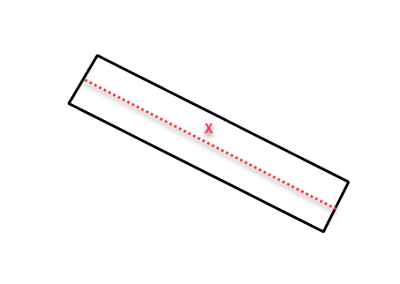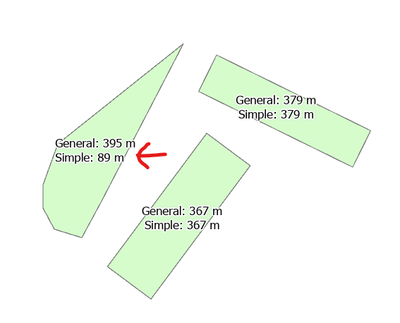# Calculate longest length of polygon

117
3
12-06-2022 09:44 AMbyMVP Frequent Contributor

I am trying to make an attribute rule that automatically calculates the length of the longest side of a polygon, but having difficulty figuring it out.

Polygon will always be a 4 sided rectangle, but may be oriented in any direction.

Does any know of a way to accomplish this as an attribute rule?

Basically, need the X value here:Thanks for any help,

R_

1 Solution

Accepted SolutionsbyMVP Regular Contributor
``````var distances = []
// get the polygon parts
var rings = Geometry(\$feature).rings
// loop over the parts
for(var r in rings) {
var ring = rings[r]
// loop over the vertices (skip first)
for(var v = 1; v < Count(ring); v++) {
Push(distances, Distance(ring[v - 1], ring[v]))
}
}
return Max(distances)``````

This will get the longest side of any polygon, no matter the part count or the form. You could simplify it by only extracting the first ring and checking only the first three vertices (giving you the two sides), but it would not make any noticeable time difference.

Have a great day!
Johannes
3 RepliesbyMVP Regular Contributor
``````var distances = []
// get the polygon parts
var rings = Geometry(\$feature).rings
// loop over the parts
for(var r in rings) {
var ring = rings[r]
// loop over the vertices (skip first)
for(var v = 1; v < Count(ring); v++) {
Push(distances, Distance(ring[v - 1], ring[v]))
}
}
return Max(distances)``````

This will get the longest side of any polygon, no matter the part count or the form. You could simplify it by only extracting the first ring and checking only the first three vertices (giving you the two sides), but it would not make any noticeable time difference.

Have a great day!
JohannesbyMVP Regular Contributor

For completeness, here's the simple expression for a rectangle:

``````var ring = Geometry(\$feature).rings
var d1 = Distance(ring, ring)
var d2 = Distance(ring, ring)
return Max(d1, d2)``````Have a great day!
JohannesbyMVP Frequent Contributor

Thank you  @JohannesLindner, this is exactly what I needed.

Only thing I see that is weird, is that it was reporting distances in meters, even though my map and data are in State Plane feet.

Documentation says 'Distance' defaults to the spatial reference of the data, but that does not seem to be the case with a calculation attribute rule.

Adding the units parameter did the trick though:

``Push(distances, Distance(ring[v - 1], ring[v], 'feet'))``

Thanks again,

R_